## Fluids

•Max4
Subscriber

Hello,

I have done a simulation which models a combustion chamber. My problem is the following:

At t=0.033s, the inlet changes from "velocity" to "wall" and the piston goes up. However, we can see that a t=0.034s, that there is no more flow in the entrance (circled in red - going from 4m/s to 0 m/s).

t=0.032s                                       t=0.033s                                    t=0.034s

How can we explain that?

Regards,

Maxime

•DrAmine
Ansys Employee

What about the velocity of the flow there at 0.033 and the length of the entrance region?

•Max4
Subscriber
•DrAmine
Ansys Employee

Might the flow already left that region. You can make a test with smaller time step size just @ 0.033 s to see what happens.

•Max4
Subscriber

The time step depends on the mesh and the velocities. i.e. the time step should be able to resolve the flow in the smallest cell.

delta t < minimum mesh size / max velocity   -> delta t < (1e-3) m / 5 m/s = 0.0002 s

I run the simulation with a change of the time step of 0.0001 s (using Events) for flow-time between 0.032 s and 0.034s. Results are as follows:

t=0,0329 s                                                             t=0,0330 s                                                                 t=0,0331 s

I´ve just calculate the Reynolds Number, and it is equal to 50 000 -> turbulent ; problem is that I ran the simulation for laminar flow. Could the problem come from the Models ?

Regards,

Maxime

•Rob
Ansys Employee

Is this a 2d case, and what's the mesh like in the inlet area? Also, what are the material properties? Please post some more images.

I'd not expect turbulence to play that big a part in the problem, but it could be a factor.

•seeta gunti
Ansys Employee

Hello,

since your inlet velocity is 5m/sec, in 0.0032sec, flow will reach 16mm. When I look at your pics, the time difference b/n the pics is 0.01sec which means that the flow has crossed 16mm and it would go 50mm. As Amine mentioned, the flow has left the inlet region.

By looking at the second attached images, I understand that you are monitoring of 1e-04 sec. I suggest to plot vectors to check the flow field. I also suggest to turn on turbulence models and check the flow filed at 1e-04 secc interval for more understanding.

•Max4
Subscriber

Hi everyone,

- yes it´s a 2D case, and here a picture of the mesh (size 1E-3m)I have run the simulation with water liquid with the default parameter of Fluent.

- The time difference between the first pics is 0.001s. At 0.033s, the inlet changes to wall and with a maximum velocity of 5 m/s. Thus the flow could just crossed 5mm which is smaller than the length of the intlet region (16mm).

I´m going to plot vectors and trying to run the simulation with a turbulence model.

Regards,

Maxime

•Max4
Subscriber

Some pictures with vectors (laminar flow). At t=0.0331, we also can see (framed in red) an area where the flow still has a speed after closing.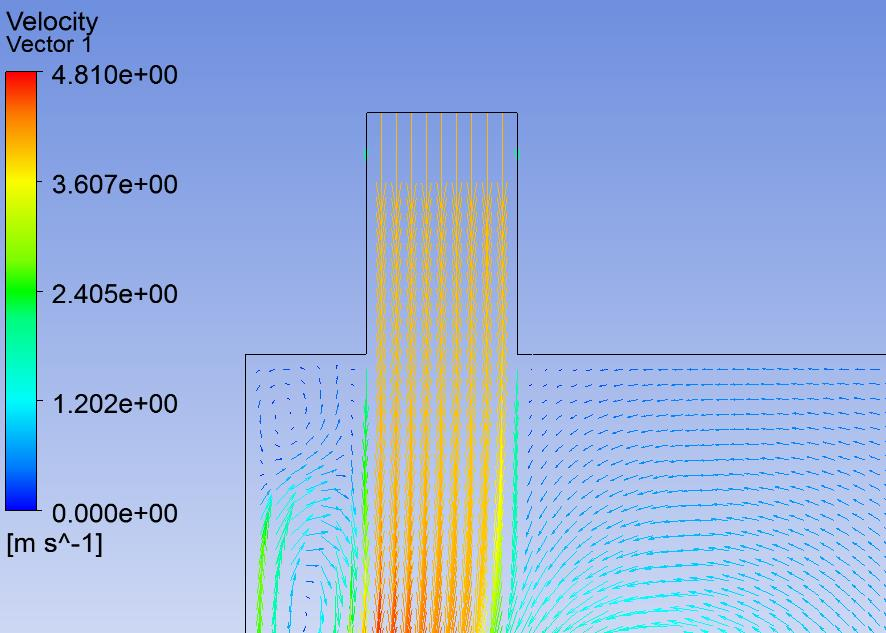t=0.0329 s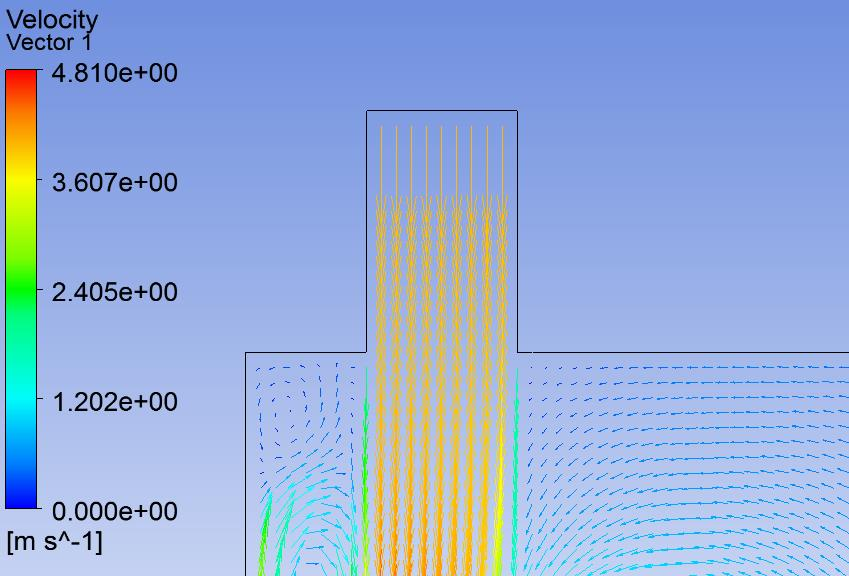t=0.0330 s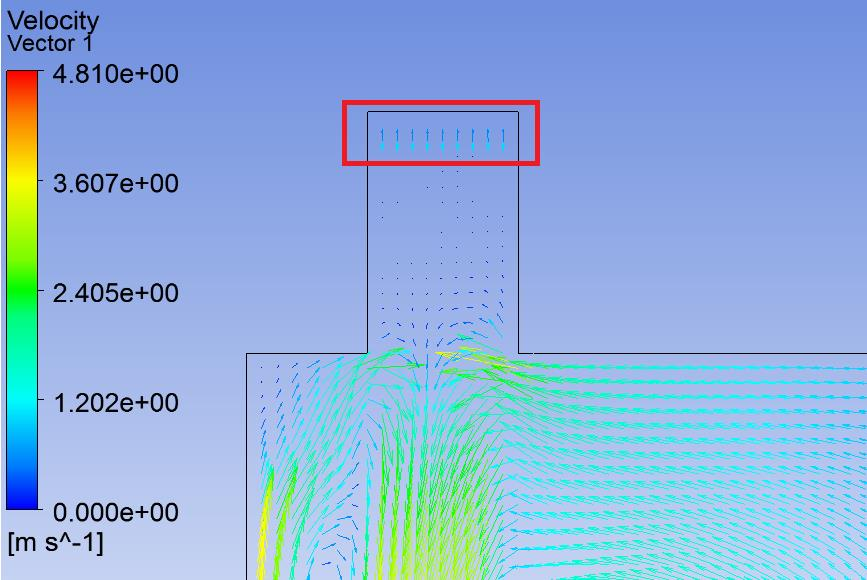t=0.0331 s

•DrAmine
Ansys Employee

Looks reasonable for me.

•Max4
Subscriber

The velocity of the flow isn´t so high to cross all or the half of the inlet region in 0.0001s. Why have I velocity vectors at the inlet after closing (framed in red) ?

Regards,

Maxime

•seeta gunti
Ansys Employee

Hi Max,

Can you turn on turbulence model and check the same velocity vectors at the same time. These results are reasonable for me.  At the time of 0.0331s, you could some recirculation entering into the inlet region but could not observe the same in 0.330sec. So please check how this bubble is moving wrt time.  With turbulence, there is definitely a change in the bubble movement.

•Max4
Subscriber

Hi,

How does it be possible that there isn´t any flow into the inlet region if the time step is 0.0001s and thus the flow could cross maximum 0.5mm?

Regards,

Maxime

•DrAmine
Ansys Employee

You have the velocity in the cells near the inlet and not at the inlet.  The results are still reasonable for me.

You can create some transformed surfaces from your inlet at tiny successive distances to monitor the overall behavior of area-weighted average of velocity or mass-flow there over the time

•Max4
Subscriber

I want to run this simulation with the standard k-epsilon model and standard wall functions.

I have calculate the intensity with this equation and Re=39722 (for fully-developed duct flow):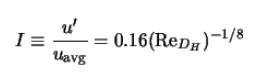=>  I= 0.043 (4.3%), it´s ok.

Then, I want to calculte k and epsilon for the Solution Controls: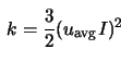=> with Uavg = 4 m/s : k=0.045 m^2/s^2 . By default, there is a value of 0.8 in Solution Controls. I think it´s an incorrect value.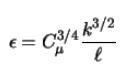=> with l=0.07*Duct_Diameter and C=0.09 (empirical constant) : epsilon = 2.24 m^2/s^3. This value seems to be incorrect, but I can´t find my error.

The values of k and epsilon are always between 0 and 1 ?

For the BC, I use Intensity and Hydraulic Diameter for the turbulence specification.

Regards,

Maxime

•DrAmine
Ansys Employee

If you want to start from fully developed conditions just set up a periodic 2d channel and then export the values of k an epsilon as well the velocity profiles into your active case.

The values of solution  controls are not used here.

The bulk velocity corresponding to mass flow rate at the boundary is the one to use to get the TKE.

•Max4
Subscriber

Thank you for your advise. So in my case, the following values of solution controls are not take into account for the simulation ?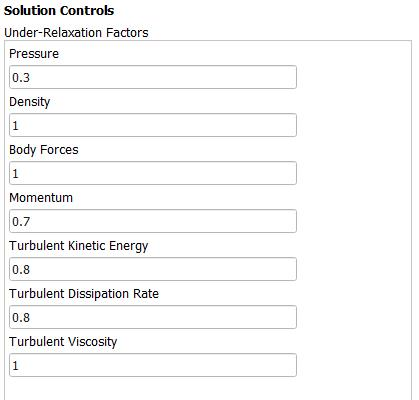Regards,

Maxime

•DrAmine
Ansys Employee

They are taken into account and implicit under-relaxation factors for the descritization of the non-linear equations. They do not affect the way you are following to get turbulence quantities.

•Max4
Subscriber

I have run a simulation with turbulence model. When I initialize, it gaves me the following values, which are the same that I have calculate.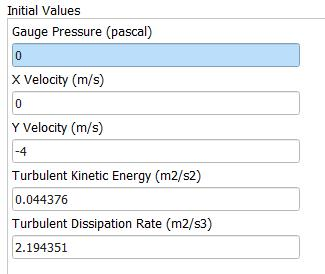Do I run the simulation with this values instead of the default values of the solution controls?

Regards,

Maxime

•DrAmine
Ansys Employee

The Solution control are not used for initialization. Check the manual to understand the meaning of under-relaxation factors.

Run the simulation with the values populated from "Initial Values".

•Max4
Subscriber

Here a good video in order to use the velocity boundary conditions from one simulation and apply it to another one :